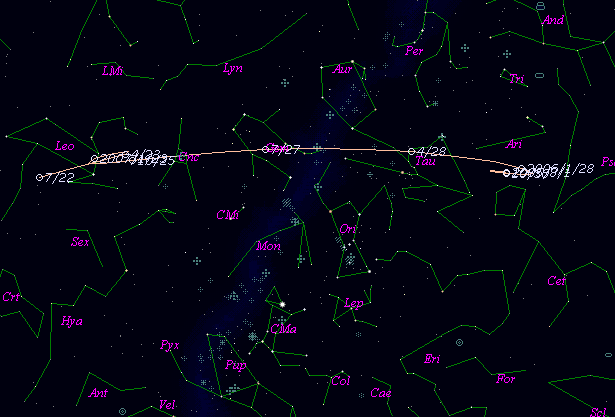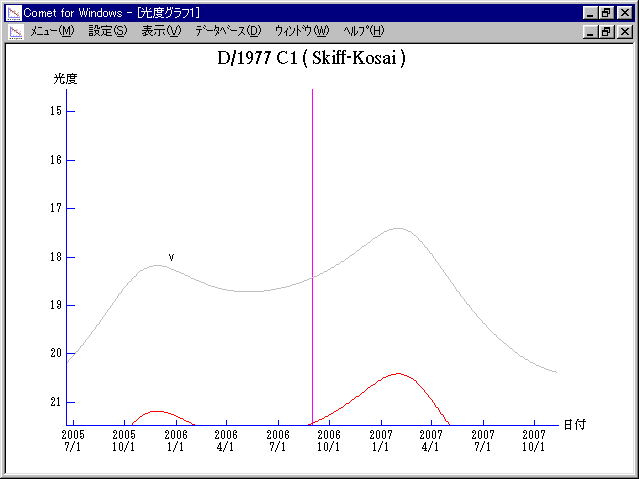# D/1977 C1 ( Skiff-Kosai ) 2006###Orbital Elements

```Perturbed ephemeris below is based on elements from MPC 11152.

Epoch = 2453960.50000 TT   T = 2453978.86738 TT
Peri. =   26.56336         a =     3.8211067 AU
Node  =   80.37021 J2000.0 e =     0.2684986
Incl. =    3.20335         q =     2.7951451 AU
```

###Finding Charts###Magnitudes Graph

```        m1 = 12 + 5 log d + 15 log r

* Gray curve is:  m1 = 9.0 + 5 log d + 15 log r
```##### The orbital elements are calculated using the Minor Planet & Comet Ephemeris Service. The charts are made with StellaNavigator Ver.2.0 for Windows (AstroArts / ASCII). The magnitudes graphs are made with Comet for Windows.